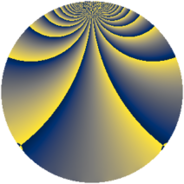# Properties

 Label 1815.2.bkLevel $1815$ Weight $2$ Character orbit 1815.bk Rep. character $\chi_{1815}(16,\cdot)$ Character field $\Q(\zeta_{55})$ Dimension $3520$ Sturm bound $528$

# Related objects

## Defining parameters

 Level: $$N$$ $$=$$ $$1815 = 3 \cdot 5 \cdot 11^{2}$$ Weight: $$k$$ $$=$$ $$2$$ Character orbit: $$[\chi]$$ $$=$$ 1815.bk (of order $$55$$ and degree $$40$$) Character conductor: $$\operatorname{cond}(\chi)$$ $$=$$ $$121$$ Character field: $$\Q(\zeta_{55})$$ Sturm bound: $$528$$

## Dimensions

The following table gives the dimensions of various subspaces of $$M_{2}(1815, [\chi])$$.

Total New Old
Modular forms 10720 3520 7200
Cusp forms 10400 3520 6880
Eisenstein series 320 0 320

## Trace form

 $$3520q - 8q^{2} + 80q^{4} - 8q^{7} + 16q^{8} - 880q^{9} + O(q^{10})$$ $$3520q - 8q^{2} + 80q^{4} - 8q^{7} + 16q^{8} - 880q^{9} + 16q^{10} + 4q^{11} + 48q^{13} + 96q^{14} + 104q^{16} + 16q^{17} + 12q^{18} - 8q^{19} + 32q^{22} + 64q^{23} + 36q^{24} + 88q^{25} - 8q^{26} - 28q^{28} - 8q^{29} - 4q^{30} + 40q^{31} - 56q^{32} - 4q^{33} - 72q^{34} - 8q^{35} + 80q^{36} + 60q^{37} + 44q^{38} - 8q^{39} + 120q^{40} + 28q^{41} - 24q^{42} + 40q^{43} - 8q^{44} - 24q^{46} + 48q^{47} - 32q^{48} + 144q^{49} - 8q^{50} + 4q^{51} + 256q^{52} + 256q^{53} + 12q^{55} - 52q^{56} - 8q^{57} + 168q^{58} - 40q^{59} - 20q^{61} + 204q^{62} - 8q^{63} + 212q^{64} - 24q^{65} - 20q^{66} + 20q^{67} + 40q^{68} - 8q^{69} + 36q^{70} - 4q^{72} + 80q^{73} + 84q^{74} + 172q^{76} + 92q^{77} + 156q^{78} + 252q^{79} - 880q^{81} + 132q^{82} + 4q^{83} + 48q^{84} - 8q^{85} + 72q^{86} + 88q^{87} + 68q^{88} + 80q^{89} - 4q^{90} + 176q^{91} + 104q^{92} - 16q^{93} + 212q^{94} + 276q^{96} + 120q^{97} + 256q^{98} + 24q^{99} + O(q^{100})$$

## Decomposition of $$S_{2}^{\mathrm{new}}(1815, [\chi])$$ into newform subspaces

The newforms in this space have not yet been added to the LMFDB.

## Decomposition of $$S_{2}^{\mathrm{old}}(1815, [\chi])$$ into lower level spaces

$$S_{2}^{\mathrm{old}}(1815, [\chi]) \cong$$ $$S_{2}^{\mathrm{new}}(121, [\chi])$$$$^{\oplus 4}$$$$\oplus$$$$S_{2}^{\mathrm{new}}(363, [\chi])$$$$^{\oplus 2}$$$$\oplus$$$$S_{2}^{\mathrm{new}}(605, [\chi])$$$$^{\oplus 2}$$